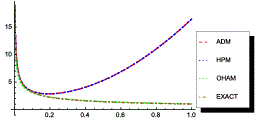Compartilhar:

ABSTRACT

In the present paper , we obtain analytical-approximate solution of Abel Volterra integral equations by using Optimal Homotopy Asymptotic method (OHAM).This approach has been compared with some other powerful and efficient methods such as He’s homotopy perturbation method (HPM) and Adomian decomposition method (ADM). This method uses simple computations with quite acceptable approximate solutions, which has close with exact solutions. The accuracy and efficiency of OHAM approach is compared and illustrated by presenting four test examples that satisfy the power of OHAM compared to ADM and HPM methods. agreement

Keywords: Nonlinear singular Volterra integral equations of Abel type, Optimal Homotopy

Compartilhar: